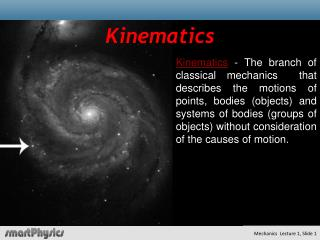DownloadDownload PresentationKinematics

# Kinematics

Télécharger la présentation## Kinematics

- - - - - - - - - - - - - - - - - - - - - - - - - - - E N D - - - - - - - - - - - - - - - - - - - - - - - - - - -
##### Presentation Transcript

1. Kinematics Kinematics - The branch of classical mechanics that describes the motions of points, bodies (objects) and systems of bodies (groups of objects) without consideration of the causes of motion.

2. Classical Mechanics Lecture 1 Today's Concepts: a) Displacement, Velocity, Acceleration b) 1-D Kinematics with constant acceleration If you haven't done Prelecture 1 yet, please do so later today

3. Main Points of Unit 1

4. Main Points of Unit 1

5. Prelecture ExampleDisplacement

6. Prelecture ExampleDisplacement

7. Displacement and Velocity in One Dimension Displacement Time taken But what about ?

8. Displacement and Velocity in One Dimension Thev(t)vs.tplot is just theslope of thex(t)vs.tplot Definition: Speed = |v(t)| Theslope of thex(t)vs.tplot is the “derivative” of x(t) w.r.t t. Limit of Dt0

9. Displacement and Velocity in One Dimension

10. Displacement as a function of velocity

11. Displacement and Velocity in one dimension • Yes • NO Are the plots shown at the left correctly related?

12. Prelecture Question 1

13. Prelecture Question 1

14. Prelecture Help Question 1

15. Prelecture Question 2

16. Prelecture Question 2

17. Clicker Question The velocity vs. time plot of some object is shown to the right. Which diagram below could be the Displacement vs. time plot for the same object? A B C

18. Which Plot? • Plot A • Plot B • Plot C • None of the above A B C

19. Clicker Question The velocity vs. time plot of some object is shown to the right. Which diagram below could be the Displacement vs. time plot for the same object? A B C

20. Acceleration

21. Checkpoint 1 For the Displacement and Velocity curves shown on the left, which is the correct plot of acceleration vs. time? A B

22. Checkpoint 1 A B Correct answer

23. Checkpoint 1-responses

24. Checkpoint 1-responses

25. Checkpoint 1

26. Acceleration https://www.smhttps://www.smartphysics.com/Course/PlaySlideshow?unitItemID=192801&enrollmentID=29042artphysics.com/Course/PlaySlideshow?unitItemID=192801&enrollmentID=29042

27. Constant Acceleration constanta(t) = a

28. Checkpoint 2 Att = 0a ball, initially at rest, starts to roll down a ramp with constant acceleration. Suppose it moves1footbetweent = 0secandt = 1 sec. How far does it move betweent = 1 secandt = 2 sec? A) 1 foot B) 2 feet C) 3 feet D) 4 feet E) 6 feet

29. Checkpoint 2 ? 1ft 4ft 9ft 16ft Att = 0a ball, initially at rest, starts to roll down a ramp with constant acceleration. Suppose it moves1footbetweent = 0secandt = 1 sec. How far does it move betweent = 1 secandt = 2 sec? A) 1 foot B) 2 feet C) 3 feet D) 4 feet E) 6 feet

30. Checkpoint 2 Responses Excellent Answer

31. Checkpoint 2 Responses Yes we do!

32. Checkpoint 2 Responses

33. Checkpoint 2 Responses

34. Checkpoint 2 Solution 3 1ft 4ft 9ft 16ft

35. Main Points of Unit 1

36. Main Points of Unit 1

37. A “real world” ramp experiment Kilometre lance http://www.kl-france.com/

38. A “real world” ramp experiment Assume constant acceleration Verbier- Mont Fort Could not find elapsed times…to check.